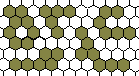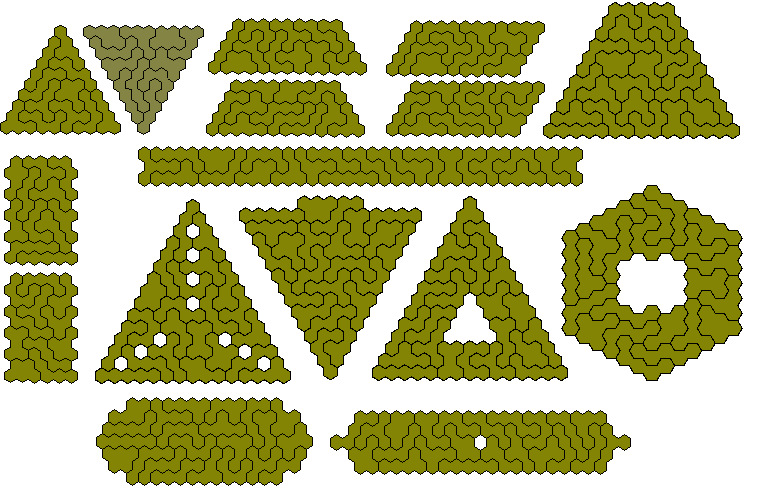PolyhexesThere are three regular polygons which tile the plane- square, equilateral triangle and regular hexagon. Thus in the same way as a square grid gives us polyominoes and an isometric grid polyiamonds we obtain polyhexes from an hexagonal (honeycomb) grid. Such a grid is shown below with five examples of polyhexes.TetrahexesIf we consider the one-sided tetrahexes then we find that there are 10. These are shown in the pattern below.Pentahexes

There are 22 pentahexes are shown below in a pattern of two 'triangles' made by Christoph M. Hoffman which appeared in Martin Gardner's 'Mathematical Games' in the Scientific American in June 1967 together with a selection of other constructions.We may also look for a figure with the maximum number of internal holes. The best known solution is with 30 holes.There are 33 one-sided pentahexes shown here in three triangles with the central triangle formed from the symmetric pieces and the other two triangles being reflections of each another..A number of symmetrical constructions are possible.A number of the figures above are in the shape of a trapezium. An a-b trapezium as shown below will contain ½b(2a+b-1) hexagons.For the one-sided pentahexes the total area is 165 and so we must have b(2a+b-1)=330 from which we get the following possibilities for trapezia - 54-3; 31-5; 25-6; 12-10; 4-15 - all of which are shown above.

Fixed Orientation Polyhexes

There are 44 tetrahexes if we do not allow rotation or reflection of the pieces.